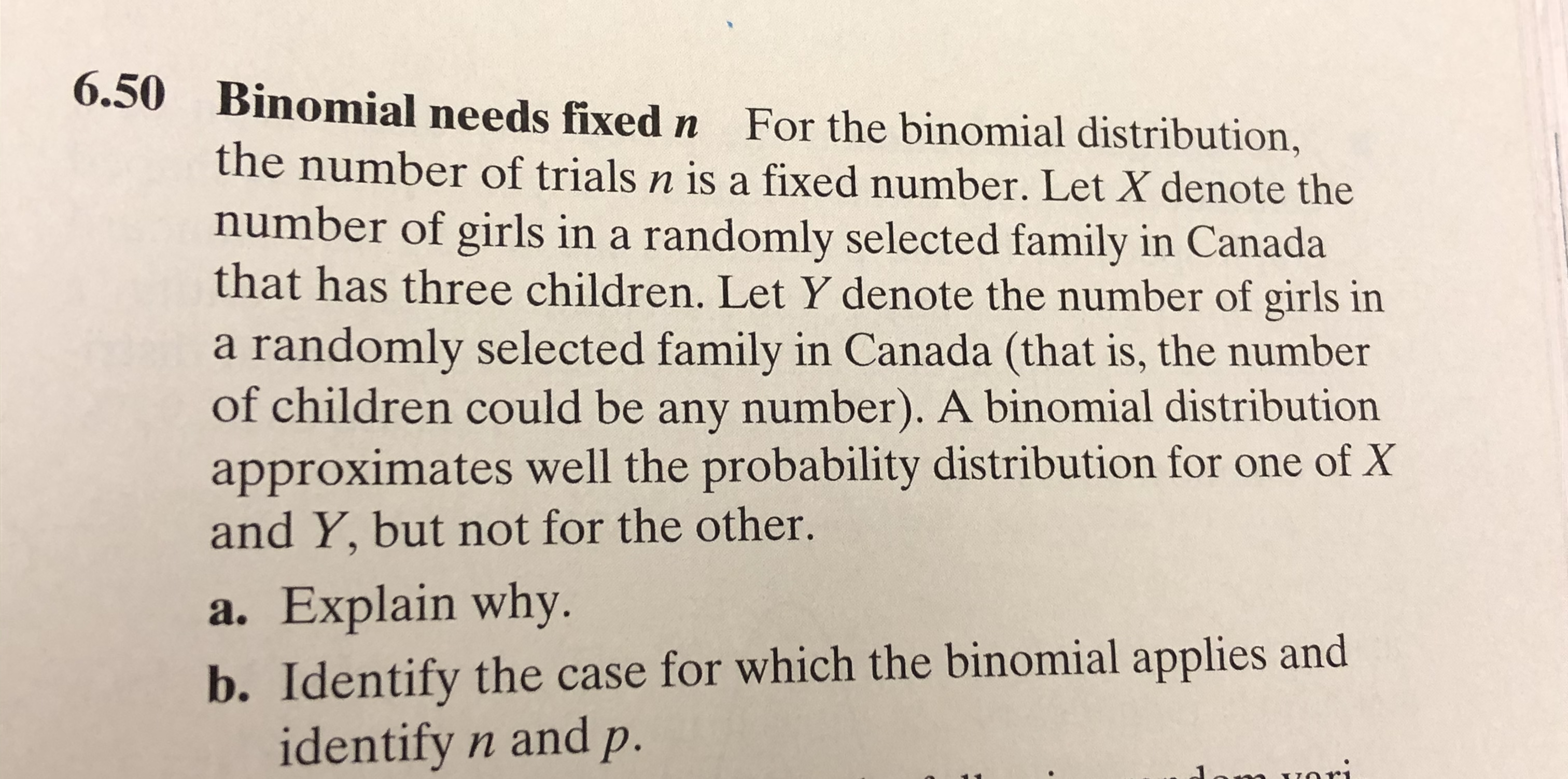# 6.50Binomial needs fixed nFor the binomial distribution,the number of trials n is a fixed number. Let X denote thenumber of girls in a randomly selected family in Canadathat has three children. Let Y denote the number of girls ina randomly selected family in Canada (that is, the numberof children could be any number). A binomial distributionapproximates well the probability distribution for one of Xand Y, but not for the other.a. Explain whyb. Identify the case for which the binomial applies andidentify n andp.m vori

Questionhelp_outlineImage Transcriptionclose6.50 Binomial needs fixed n For the binomial distribution, the number of trials n is a fixed number. Let X denote the number of girls in a randomly selected family in Canada that has three children. Let Y denote the number of girls in a randomly selected family in Canada (that is, the number of children could be any number). A binomial distribution approximates well the probability distribution for one of X and Y, but not for the other. a. Explain why b. Identify the case for which the binomial applies and identify n andp. m vori fullscreen
check_circle

Step 1

From the given information,

For binomial distribution, the number of trials n are always constant or fixed.

So,

X = number of girls in a randomly selected fam...

### Want to see the full answer?

See Solution

#### Want to see this answer and more?

Solutions are written by subject experts who are available 24/7. Questions are typically answered within 1 hour.*

See Solution
*Response times may vary by subject and question.
Tagged in

### Statistics